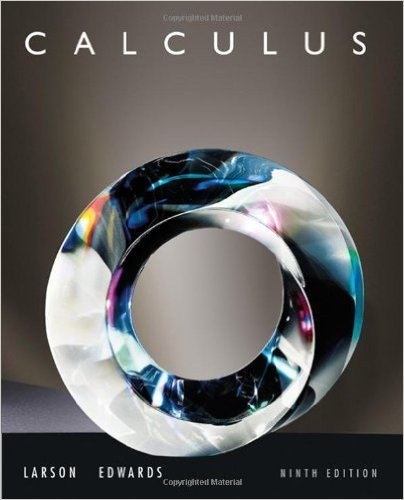×
×

# Solutions for Chapter 3.8: Newtons Method## Full solutions for Calculus | 9th Edition

ISBN: 9780547167022Solutions for Chapter 3.8: Newtons Method

Solutions for Chapter 3.8
4 5 0 330 Reviews
16
1
##### ISBN: 9780547167022

Since 46 problems in chapter 3.8: Newtons Method have been answered, more than 63260 students have viewed full step-by-step solutions from this chapter. This textbook survival guide was created for the textbook: Calculus , edition: 9. This expansive textbook survival guide covers the following chapters and their solutions. Chapter 3.8: Newtons Method includes 46 full step-by-step solutions. Calculus was written by and is associated to the ISBN: 9780547167022.

Key Calculus Terms and definitions covered in this textbook
• Angle of depression

The acute angle formed by the line of sight (downward) and the horizontal

• Blind experiment

An experiment in which subjects do not know if they have been given an active treatment or a placebo

• Bounded interval

An interval that has finite length (does not extend to ? or -?)

• Convergence of a sequence

A sequence {an} converges to a if limn: q an = a

• Course

See Bearing.

• Difference identity

An identity involving a trigonometric function of u - v

• Dihedral angle

An angle formed by two intersecting planes,

• Direction angle of a vector

The angle that the vector makes with the positive x-axis

• Dot product

The number found when the corresponding components of two vectors are multiplied and then summed

• Inequality

A statement that compares two quantities using an inequality symbol

• Initial value of a function

ƒ 0.

• NDER ƒ(a)

See Numerical derivative of ƒ at x = a.

• Numerical derivative of ƒ at a

NDER f(a) = ƒ1a + 0.0012 - ƒ1a - 0.00120.002

• One-to-one rule of exponents

x = y if and only if bx = by.

• Pie chart

See Circle graph.

• Sample standard deviation

The standard deviation computed using only a sample of the entire population.

• Symmetric difference quotient of ƒ at a

ƒ(x + h) - ƒ(x - h) 2h

• Symmetric property of equality

If a = b, then b = a

• Venn diagram

A visualization of the relationships among events within a sample space.

• Vertex form for a quadratic function

ƒ(x) = a(x - h)2 + k

×